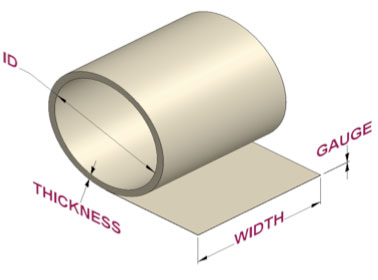# Coil Calculator

Use the Coil Calculator to estimate the amount of metal on a coil. Select from steel, aluminum, or copper. Select your gauge and fill in the measurements to determine the square footage, the lineal footage and weight.The values calculated by the coil calculator are just estimates and as such CMG can assume no liability with the use of this calculator.

Gauge:
(steel gauge)
ID:
(inch)
Width:
(inch)
Thickness:
(inch)

Sq. FT.:
0
Linear FT.:
0
Weight (lb.):
0

Gauge:
(aluminum gauge)
ID:
(inch)
Width:
(inch)
Thickness:
(inch)

Sq. FT.:
0
Linear FT.:
0
Weight (lb.):
0

Gauge:
(copper gauge)
ID:
(inch)
Width:
(inch)
Thickness:
(inch)

Sq. FT.:
0
Linear FT.:
0
Weight (lb.):
0